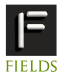# SCIENTIFIC PROGRAMS AND ACTIVITIESOctober  4, 2023## Ottawa-Carleton Graph Theory Workshop May 11-13, 2008

### School of Mathematics and Statistics Carleton University, Ottawa

Supported byOrganizers:Kevin Cheung <kcheung(at) math.carleton.ca>
Mateja Sajna <msajna (at) uottawa.ca>
Jason Gao <zgao(at)math.carleton.ca>

### Abstracts

Charlie Colbourn, Arizona State University
Graph Decompositions and Optimal Grooming

Given a multigraph $G=(V,E)$ and a set of graphs ${\cal H} = \{H_1,\dots,H_s\}$, an $\cal H$-decomposition of $G$ is a partition of its edge set into subgraphs, each of which is isomorphic to a member of $\cal H$. Graph decompositions have been widely studied in graph theory and in combinatorial design theory. Indeed in the very special case when ${\cal H}$ contains only the complete graph $K_k$ and $G \is K_v$, a decomposition is a balanced incomplete block design (with $\lambda=1$). In order to model the assignment of traffic to wavelengths in an optical ring network, this definition has been extended to associate a cost with each graph $H_i$, and to ask for a decomposition of minimum total cost.

Of particular interest is the case when $G \is K_v$ and $\cal H$ contains all simple graphs on at most $C$ edges. Then the decomposition is called a $C$-grooming. Taking the cost of each $H_i$ to be its number of vertices of nonzero degree, a $C$-grooming of minimum cost yields a wavelength assignment in an optical ring that incurs the least hardware cost.

We first outline the almost complete determination of minimum cost $C$-groomings for $1 \leq C \leq 6$ using techniques from graph theory and design theory, and report on recent results for $C \in \{7,8,9\}$. We examine graph- and design-theoretic constructions to obtain upper bounds on the cost, and develop lower bounds via an interesting use of linear programming duality.
A 'two-period' variant of grooming in which a $C$-grooming on all nodes must induce a $C'$-grooming with $C' < C$ is examined in the cases when $1\leq C \leq 4$, where complete determinations of the minimum cost have been completed. The talk focuses on general design- and graph-theoretic techniques that apply, and no background in optical networking is assumed.

Michel X. Goemans, Massachusetts Institute of Technology
Minimum Bounded Degree Spanning Trees

In this talk, I will consider the minimum spanning tree problem under the additional restriction that all degrees of the spanning
tree must be at most a given value $k$. I will describe two approaches, one by myself and one by Mohit Singh and Lap Chi
Lau. These results show that one can efficiently find a spanning tree of maximum degree at most $k+2$, or even at most $k+1$ for the second approach, whose cost is at most the cost of the optimum spanning tree of maximum degree $k$. This is best possible, as the problem of just deciding whether a graph has a spanning tree of maximum degree $k$ is NP-complete.

The first approach uses a sequence of simple algebraic, polyhedral and combinatorial arguments, and relies on uncrossing, polyhedral
characterizations, matroid intersection and graph orientation. The second approach also uses uncrossing and is a prime example of
iterative relaxation, a new technique extending Jain's iterative rounding.

Penny Haxell, University of Waterloo
Scarf's Lemma and the Stable Paths Problem

We address a question in graphs called the stable paths problem, which is an abstraction of a network routing problem concerning the Border Gateway Protocol (BGP). The main tool we use is Scarf's Lemma, an important result from game theory. This talk will describe Scarf's Lemma and how it is related to other results more familiar to combinatorialists, and then will explain its implications for the stable paths problem.

Bruce Reed, McGill University
Graph Colouring a la Chvatal.

A natural approach to solving combinatorial optimization problems is to formulate them as Integer Linear Programs, solve the
fractional relaxation, and attempt to use this solution to solve the Integer Linear Program. We discuss graph colouring from this perspective. In doing so, we consider a three pronged approach which combines polyhedral combinatorics, structural decompositions, and the probabilistic method. I learnt all three techniques as a young lad from Vasek Chvatal.

Xingxing Yu , Georgia Institute of Technology
On judicious partitions of graphs

Judicious partition problems ask for partitions of the vertex set of a graphs so that several quantities are optimized simultaneously. I will discuss several judicious partition problems of Bollobas and Scott, and present our recent results on these problems.
This is joint work with Baogang Xu.